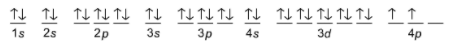# Problem: Write the corresponding electron configuration for the following pictorial representation.Give the full electron configuration (do not use the noble gas abbreviation). Name the element, assuming that the configuration describes a neutral atom.

###### FREE Expert Solution
84% (291 ratings)
###### FREE Expert Solution

We’re being asked to determine the element and electron configuration based on the given orbital diagram:Recall that:

s–subshell can hold a maximum of 2 electrons

p–subshell can hold a maximum of 6 electrons

d–subshell can hold a maximum of 10 electrons

84% (291 ratings)###### Problem Details

Write the corresponding electron configuration for the following pictorial representation.

Give the full electron configuration (do not use the noble gas abbreviation).

Name the element, assuming that the configuration describes a neutral atom.What scientific concept do you need to know in order to solve this problem?

Our tutors have indicated that to solve this problem you will need to apply the Orbital Diagrams concept. If you need more Orbital Diagrams practice, you can also practice Orbital Diagrams practice problems.

What is the difficulty of this problem?

Our tutors rated the difficulty ofWrite the corresponding electron configuration for the follo...as low difficulty.

How long does this problem take to solve?

Our expert Chemistry tutor, Sabrina took 3 minutes and 41 seconds to solve this problem. You can follow their steps in the video explanation above.

What professor is this problem relevant for?

Based on our data, we think this problem is relevant for Professor Wallace's class at LEHMAN-CUNY.